# (2) Define the permutations a and x in the group S5 by 1 2 3 4...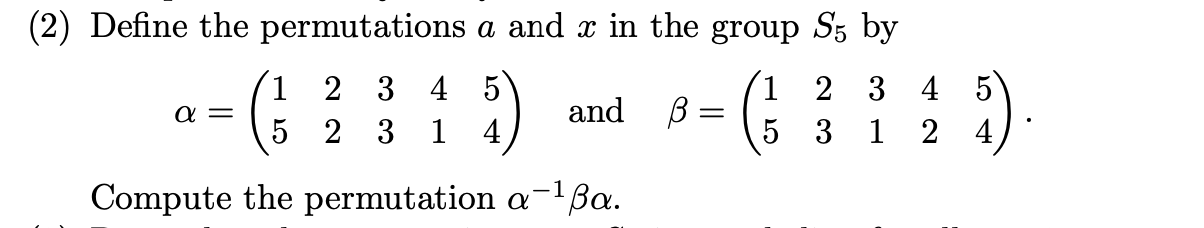(2) Define the permutations a and x in the group S5 by 1 2 3 4 5 2 3 4 and B 5 2 3 5 3 1 2 1 a = 5 = (5 1 Compute the permutation a-1 Ba.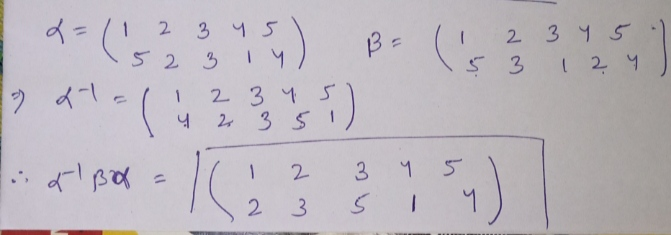I use products of permutations to solve this problem

##### Add Answer of: (2) Define the permutations a and x in the group S5 by 1 2 3 4...
Similar Homework Help Questions
• ### The following questions pertain to permutations in S8 (a) Decompose the permutation (1 2 3 4...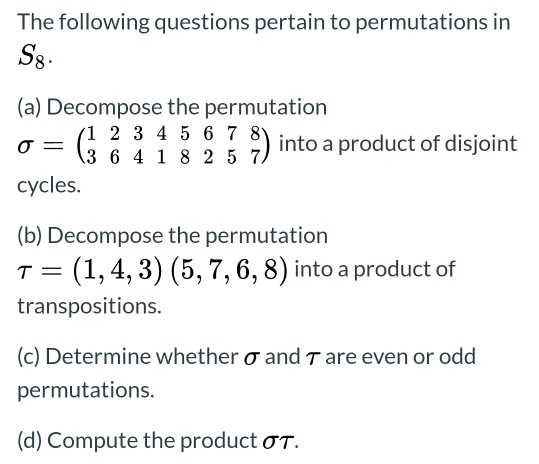The following questions pertain to permutations in S8 (a) Decompose the permutation (1 2 3 4 5 6 7 %) into a product of disjoint 13 6 4 1 8 2 5 7 cycles. = (b) Decompose the permutation T= (1,4, 3) (5,7,6,8) into a product of transpositions. (c) Determine whether o and T are even or odd permutations. (d) Compute the product OT.

• ### 2. Consider the permutations a (123)(45) and b (2543) in the symmetric group S (a) Compute the co...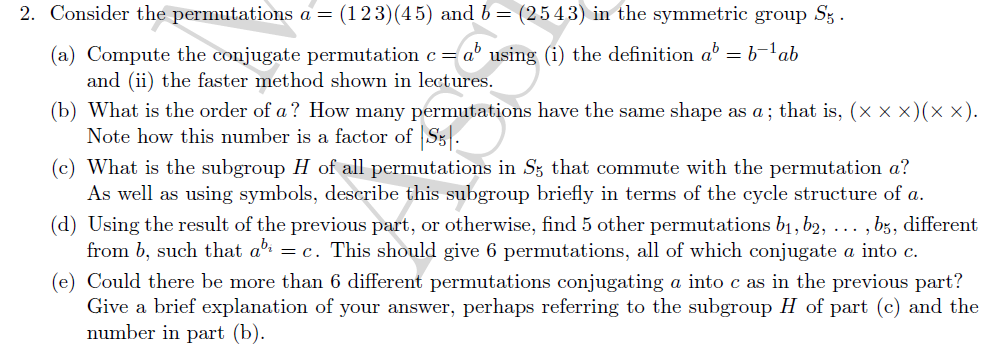2. Consider the permutations a (123)(45) and b (2543) in the symmetric group S (a) Compute the conjugate permutation ca using (i) the definition a-b ab (b) What is the order of a? How many permutations have the same shape as a; that is, (x x x)(x x). (c) What is the subgroup H of all permutations in Ss that commute with the permutation a? d) Using the result of the previous part, or otherwise, find 5 other permutations bi,...

• ### (3) (20 points) The following questions pertain to permutations in Sg. (a) Decompose the permutation o...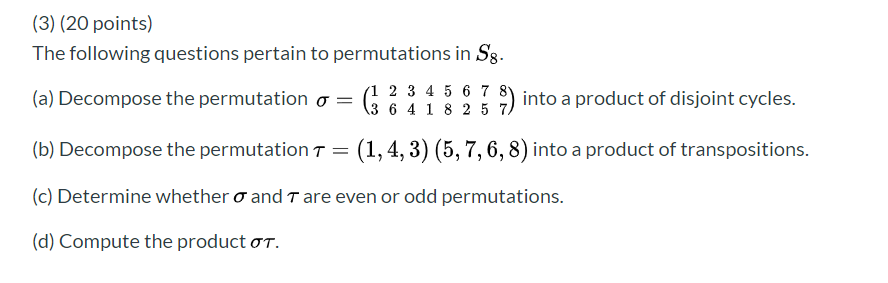(3) (20 points) The following questions pertain to permutations in Sg. (a) Decompose the permutation o (1 2 3 4 5 6 7 %) into a product of disjoint cycles. (3 6 4 1 8 2 5 7 (b) Decompose the permutation t = (1,4,3) (5,7,6,8) into a product of transpositions. (c) Determine whether o and T are even or odd permutations. (d) Compute the product ot.

• ### ASAP (3) (20 points) The following questions pertain to permutations in Sg. (a) Decompose the permutation...ASAP (3) (20 points) The following questions pertain to permutations in Sg. (a) Decompose the permutation o = (1 2 3 4 5 6 7 8) into a product of disjoint cycles. 3 6 4 1 8 2 5 (b) Decompose the permutation T = (1,4, 3) (5,7,6,8) into a product of transpositions. (c) Determine whether o and T are even or odd permutations. (d) Compute the productot.

• ### This is all about abstract algebra of permutation group. 3. Consider the following permutations in S 6 5 3 489721)' 18 73 2 6 4 59 (a) Express σ and τ as a product of disjoint cycles. (b) C...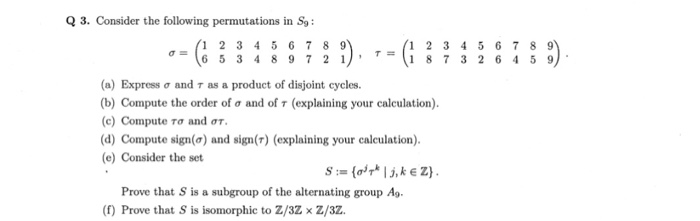This is all about abstract algebra of permutation group. 3. Consider the following permutations in S 6 5 3 489721)' 18 73 2 6 4 59 (a) Express σ and τ as a product of disjoint cycles. (b) Compute the order of σ and of τ (explaining your calculation). (c) Compute Tơ and στ. (d) Compute sign(a) and sign(T) (explaining your calculation) e) Consider the set Prove that S is a subgroup of the alternating group Ag (f) Prove that...

• ### (3) (20 points) The following questions pertain to permutations in Sg. (a) Decompose the permutation or...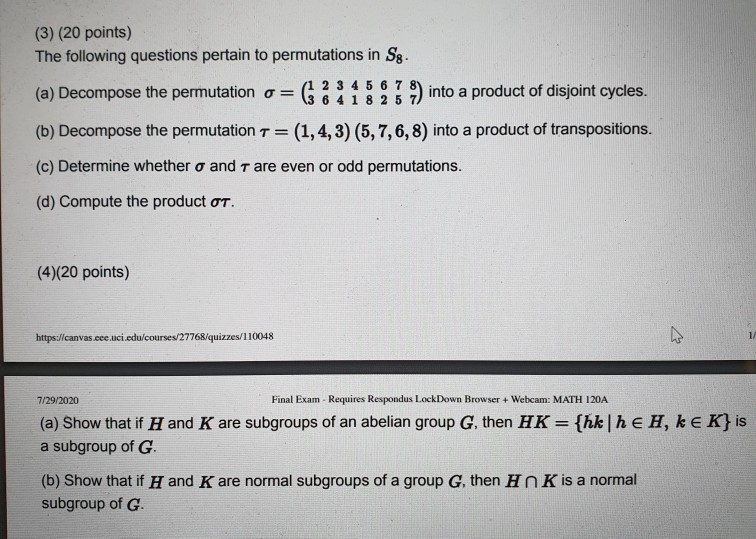(3) (20 points) The following questions pertain to permutations in Sg. (a) Decompose the permutation or 1 2 3 4 5 6 7 8) into a product of disjoint cycles. 3 6 4 1 8 2 5 (b) Decompose the permutation T= (1,4, 3) (5,7,6,8) into a product of transpositions. (c) Determine whether o and T are even or odd permutations. (d) Compute the product OT. (4)(20 points) https://canvas.coe.uci.edu/courses/27768/quizzes/110048 1. 7/29/2020 Final Exam - Requires Respondus LockDown Browser + Webcam:...

• ### (3) (20 points) The following questions pertain to permutations in Sg. (a) Decompose the permutation o=...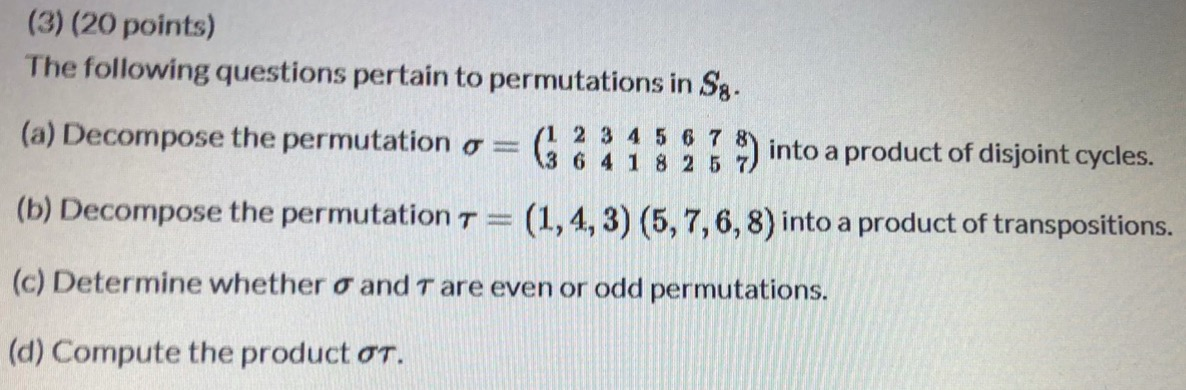(3) (20 points) The following questions pertain to permutations in Sg. (a) Decompose the permutation o= (1 2 3 4 5 6 7 (3 6 4 1 8 25 ) into a product of disjoint cycles. (b) Decompose the permutation t = (1,4, 3) (5,7,6,8) into a product of transpositions. (c) Determine whether o and Tare even or odd permutations. (d) Compute the product ot.

• ### Q= II. Permutations. Consider the following permutations in Sg: 1 2 3 4 5 6 7...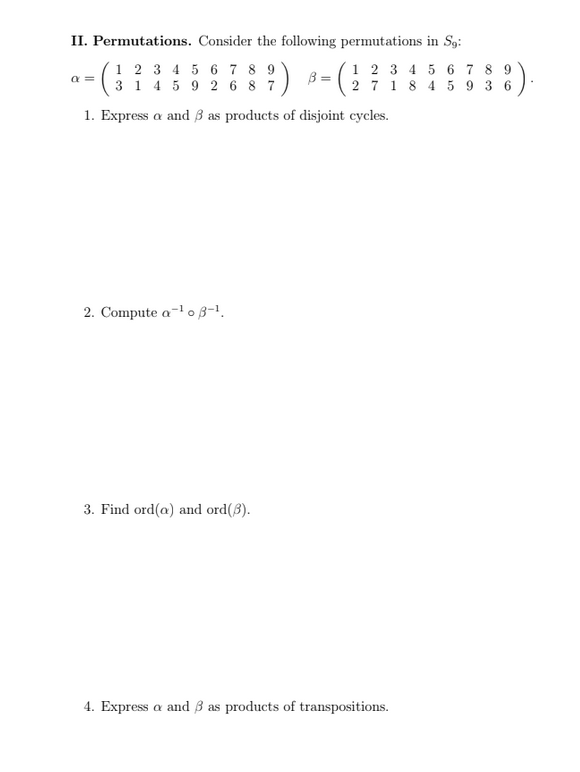Q= II. Permutations. Consider the following permutations in Sg: 1 2 3 4 5 6 7 8 9 3 1 4 5 9 2 6 8 7 2 7 1 8 4 5 9 3 6 1. Express a and B as products of disjoint cycles. 2. Compute a-108-1 3. Find ord(a) and ord(B). 4. Express a and B as products of transpositions.

• ### 6. Compute the orders of the permutations (2 1 4 6 3), (1 2)(3 4 5)...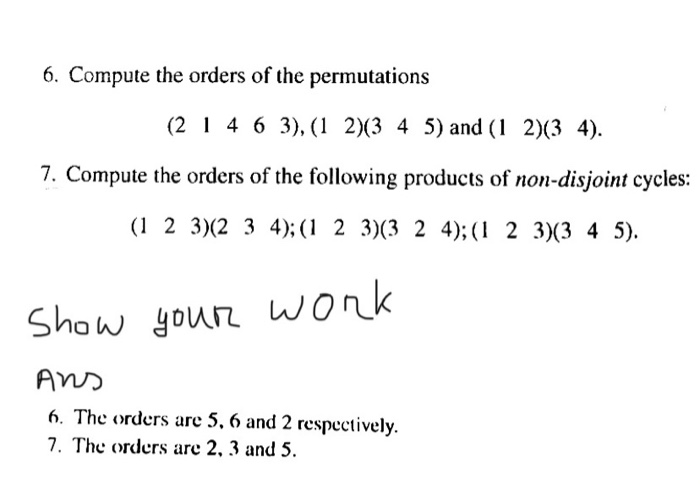6. Compute the orders of the permutations (2 1 4 6 3), (1 2)(3 4 5) and (1 2)(34). 7. Compute the orders of the following products of non-disjoint cycles: (1 2 3)(2 3 4);(1 2 3)(3 2 4);(1 2 3)(3 4 5). Show your work Ans 6. The orders are 5, 6 and 2 respectively. 7. The orders are 2, 3 and 5.

• ### 4. Determine the disorder of the permutation (1 3 5 6 2) in S6. Write (1 3 5 6 2) as a product of...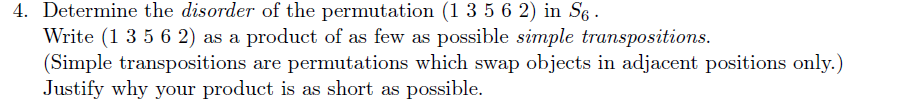4. Determine the disorder of the permutation (1 3 5 6 2) in S6. Write (1 3 5 6 2) as a product of as few as possible simple transpositions. (Simple transpositions are permutations which swap objects in adjacent positions only.) Justify why your product is as short as possible. 4. Determine the disorder of the permutation (1 3 5 6 2) in S6. Write (1 3 5 6 2) as a product of as few as possible simple transpositions....

Free Homework App